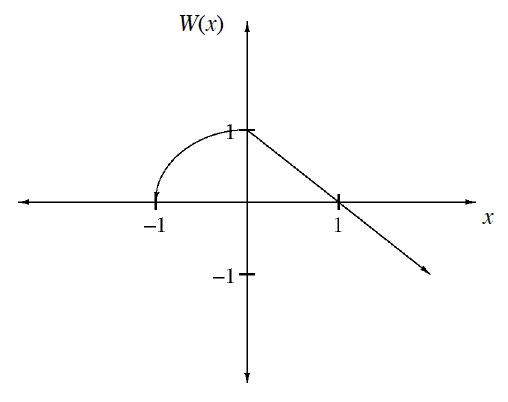### Home > CCA2 > Chapter 7 > Lesson 7.1.2 > Problem7-31

7-31.

A function $W(x)$ is sketched at right.

1. Make your own copy of the graph, and then sketch the graph of the inverse of $W(x)$.

Reflect the graph over the line $y=x$.

2. Is the inverse a function? Explain.

For every $x$-value, is there only one corresponding $y$-value?